# Ovoid

## Ovoids in-dimensional projective space.

Letdenote the-dimensional projective space over the Galois field of order. An ovoid in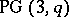is a set of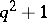points, no three of which are collinear. (Note that in [a2] and some other older publications, an ovoid is called an ovaloid.) If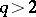, then an ovoid is a maximum-sized set of points, no three collinear, but in the casethe complement of a plane is a set ofpoints, no three collinear. More information about ovoids can be found in [a2] and [a5]. The survey paper [a4] gives further details, especially regarding more recent work, with the exception of the recent (1998) result in [a1].

The only known ovoids in(as of 1998) are the elliptic quadrics (cf. also Quadric), which exist for all, and the Tits ovoids, which exist for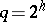where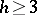is odd. There is a single orbit of elliptic quadrics under the homography group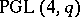, and one can take as a representative the set of points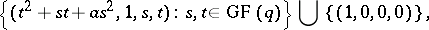whereis irreducible over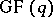. The stabilizer inof an elliptic quadric is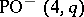, acting-transitively on its points. There is a single orbit of Tits ovoids under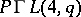, a representative of which is given by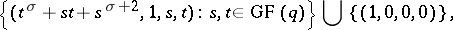where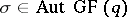is such that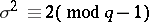. The stabilizer inof a Tits ovoid is the Suzuki group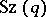acting-transitively on its points. A plane inmeets an ovoid in either a single point or in thepoints of an oval. It is worth noting that in the case of an elliptic quadric, this oval is a conic while in the case of a Tits ovoid it is a translation oval with associated automorphism.

The classification of ovoids inis of great interest, particularly in view of the number of related structures, such as Möbius planes, symplectic polarities, linear complexes, generalized quadrangles, unitals, maximal arcs, translation planes, and ovals.

Ifis odd, then every ovoid is an elliptic quadric, but the classification problem foreven has been resolved only (as of 1998) for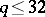, with the caseinvolving some computer work. These classifications rely on the classification of ovals in the projective planes over fields of order up to. On the other hand, there are several characterization theorems known, normally in terms of an assumption on the nature of the plane sections. One of the strongest results in this direction states that an ovoid with at least one conic among its plane sections must be an elliptic quadric. Similarly, it is known that an ovoid which admits a pencil oftranslation ovals is either an elliptic quadric or a Tits ovoid.

## Ovoids in generalized polygons.

An ovoid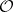in a generalized-gon(cf. also Polygon) is a set of mutually opposite points (henceis even) such that every elementofis at distance at mostfrom at least one element of. One connection between ovoids inand ovoids in generalized-gons is that just as polarities of projective spaces sometimes give rise to ovoids, polarities of generalized-gons produce ovoids. Further, every ovoid of the classical symplectic generalized quadrangle (-gon; cf. also Quadrangle) usually denoted by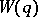,even, is an ovoid ofand conversely. It is worth noting in this context that a Tits ovoid inis the set of all absolute points of a polarity of,andodd. There are many ovoids known (1998) for most classes of generalized quadrangles, with useful characterization theorems. For the details and a survey of existence and characterization results for ovoids in generalized-gons, see [a6] and especially [a7].

## Ovoids in finite classical polar spaces.

An ovoidin a finite classical polar space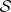of rankis a set of points ofwhich has exactly one point in common with every generator of. Again, there are connections between ovoids in polar spaces and ovoids in generalized-gons. For example, ifdenotes the classical generalized hexagon (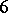-gon) of orderembedded on the non-singular quadric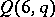, then a set of pointsis an ovoid ofif and only if it is an ovoid of the classical polar space. The existence problem for ovoids has been settled for most finite classical polar spaces, see [a3] or [a5] for a survey which includes a list of the open cases (as of 1998).

How to Cite This Entry:
Ovoid. C.M. O'Keefe (originator), Encyclopedia of Mathematics. URL: http://encyclopediaofmath.org/index.php?title=Ovoid&oldid=15585
This text originally appeared in Encyclopedia of Mathematics - ISBN 1402006098